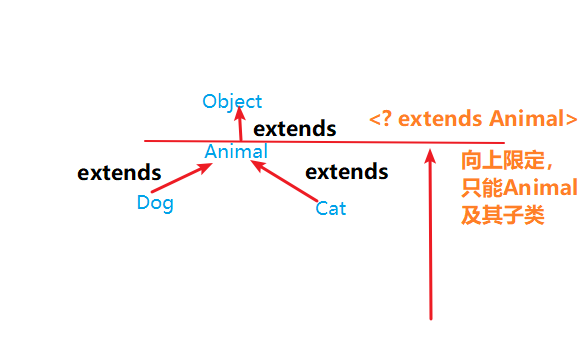java# Java 泛型详解(超详细的java泛型方法解析)

### 1. 为什么使用泛型

• 来看一个经典案例：
```public static void main(String[] args) {
//测试一下泛型的经典案例
ArrayList arrayList = new ArrayList();
for (int i = 0; i < arrayList.size(); i++) {
//需求：打印每个字符串的长度,就要把对象转成String类型
String str = (String) arrayList.get(i);
System.out.println(str.length());
}
}
```

Exception in thread "main" java.lang.ClassCastException: java.lang.Integer cannot be cast to java.lang.String

```ArrayList<String> arrayList = new ArrayList<>();
```

## 3. 使用泛型的好处

• 避免了类型强转的麻烦。
• 它提供了编译期的类型安全，确保在泛型类型（通常为泛型集合）上只能使用正确类型的对象，避免了在运行时出现ClassCastException。

## 4. 泛型的使用

### 4.1 泛型类

• 泛型类的定义格式：

```/**
* @param <T> 这里解释下<T>中的T:
*           此处的T可以随便写为任意标识，常见的有T、E等形式的参数表示泛型
*           泛型在定义的时候不具体，使用的时候才变得具体。
*           在使用的时候确定泛型的具体数据类型。即在创建对象的时候确定泛型。
*/
public class GenericsClassDemo<T> {
//t这个成员变量的类型为T,T的类型由外部指定
private T t;
//泛型构造方法形参t的类型也为T，T的类型由外部指定
public GenericsClassDemo(T t) {
this.t = t;
}
//泛型方法getT的返回值类型为T，T的类型由外部指定
public T getT() {
return t;
}
}
```

```public class GenericsClassDemo<String> {
private String t;
public GenericsClassDemo(String t) {
this.t = t;
}
public String getT() {
return t;
}
}
```

`Generic<Integer> genericInteger = new Generic<Integer>(666);`

• 注意： 定义的泛型类，就一定要传入泛型类型实参么？

### 4.2 泛型方法

• 定义格式：

```/**
*
* @param t 传入泛型的参数
* @param <T> 泛型的类型
* @return T 返回值为T类型
* 说明：
*   1）public 与 返回值中间<T>非常重要，可以理解为声明此方法为泛型方法。
*   2）只有声明了<T>的方法才是泛型方法，泛型类中的使用了泛型的成员方法并不是泛型方法。
*   3）<T>表明该方法将使用泛型类型T，此时才可以在方法中使用泛型类型T。
*   4）与泛型类的定义一样，此处T可以随便写为任意标识，常见的如T、E等形式的参数常用于表示泛型。
*/
public <T> T genercMethod(T t){
System.out.println(t.getClass());
System.out.println(t);
return t;
}
```

```public static void main(String[] args) {
GenericsClassDemo<String> genericString  = new GenericsClassDemo("helloGeneric"); //这里的泛型跟下面调用的泛型方法可以不一样。
String str = genericString.genercMethod("hello");//传入的是String类型,返回的也是String类型
Integer i = genericString.genercMethod(123);//传入的是Integer类型,返回的也是Integer类型
}
```

class java.lang.String

hello

class java.lang.Integer
123

### 4.3 泛型接口

• 定义格式
`修饰符 interface接口名<代表泛型的变量> {  }`

```/**
* 定义一个泛型接口
*/
public interface GenericsInteface<T> {
}
```

• 1、定义类时确定泛型的类型
```public class GenericsImp implements GenericsInteface<String> {
@Override
System.out.println("设置了泛型为String类型");
}
}
```
• 2、始终不确定泛型的类型，直到创建对象时，确定泛型的类型
```public class GenericsImp<T> implements GenericsInteface<T> {
@Override
System.out.println("没有设置类型");
}
}
```

```public class GenericsTest {
public static void main(String[] args) {
GenericsImp<Integer> gi = new GenericsImp<>();
}
}
```

## 5. 泛型通配符

### 5.1 通配符基本使用

```// ？代表可以接收任意类型
// 泛型不存在继承、多态关系,泛型左右两边要一样
//ArrayList<Object> list = new ArrayList<String>();这种是错误的
//泛型通配符?:左边写<?> 右边的泛型可以是任意类型
ArrayList<?> list1 = new ArrayList<Object>();
ArrayList<?> list2 = new ArrayList<String>();
ArrayList<?> list3 = new ArrayList<Integer>();
```

```public static void main(String[] args) {
ArrayList<Integer> list1 = new ArrayList<Integer>();
test(list1);
ArrayList<String> list2 = new ArrayList<String>();
test(list2);
}
public static void test(ArrayList<?> coll){
}
```

### 5.2 通配符高级使用

• 格式` 类型名称 <? extends 类 > 对象名称`
• 意义`只能接收该类型及其子类`

• 格式： `类型名称 <? super 类 > 对象名称`
• 意义： `只能接收该类型及其父类型`

```class Animal{}//父类
class Dog extends Animal{}//子类
class Cat extends Animal{}//子类
```

```//        ArrayList<? extends Animal> list = new ArrayList<Object>();//报错
ArrayList<? extends Animal> list2 = new ArrayList<Animal>();
ArrayList<? extends Animal> list3 = new ArrayList<Dog>();
ArrayList<? extends Animal> list4 = new ArrayList<Cat>();
```• 我们再来看看泛型的下限`<? super 类 >：`
```        ArrayList<? super Animal> list5 = new ArrayList<Object>();
ArrayList<? super Animal> list6 = new ArrayList<Animal>();
//        ArrayList<? super Animal> list7 = new ArrayList<Dog>();//报错
//        ArrayList<? super Animal> list8 = new ArrayList<Cat>();//报错
```• 一般泛型的上限和下限也是用来参数的传递：

```public static void main(String[] args) {
Collection<Integer> list1 = new ArrayList<Integer>();
Collection<String> list2 = new ArrayList<String>();
Collection<Number> list3 = new ArrayList<Number>();
Collection<Object> list4 = new ArrayList<Object>();
getElement(list1);
getElement(list2);//报错
getElement(list3);
getElement(list4);//报错
getElement2(list1);//报错
getElement2(list2);//报错
getElement2(list3);
getElement2(list4);
}
// 泛型的上限：此时的泛型?，必须是Number类型或者Number类型的子类
public static void getElement1(Collection<? extends Number> coll){}
// 泛型的下限：此时的泛型?，必须是Number类型或者Number类型的父类
public static void getElement2(Collection<? super Number> coll){}
118060630```